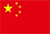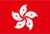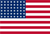Scanning QR Code• Online Inquiry

+
Congratulations
You've successfully got advanced quotes worth 5000 HKD
• Home
• Quotes
• News
• Community
• Learn
• Help Center
• Language
•••EN

# Time-weighted rate of return

Example:Rate of return

With reference to the rate of return, the time-weighted calculation rule is used to calculate the rate of return during the investment period (from April 18, 2016 to the previous trading day).

Time-weighted rate of return=[(1+R1)×(1+R2)×(1+R3)…(1+Rn)-1]×100%, Rn is the daily rate of return at time Tn.

Yield on the day = (total investment this time - total investment last time - this deposit + this withdrawal - this entry + this shipment) / (total investment last time + this deposit + this shipment)

Taking into account the transfer of funds and stocks, it will magnify the calculation of the return on the day, so it is not included in the calculation of the denominator of the return on the day.

For example, on January 1,2017, the total investment amount of A is ¥10,000, the return on that day is ¥1,000, and the return on that day is 10% (1,000/10,000). If A withdraws ¥5,000 that day, the return on that day is still 10%.

Back to the Top